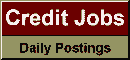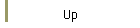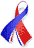DefaultRisk.com the web's biggest credit risk modeling resource.doi> search: A or B Cited by these papersRelated articlesAlternative sources Search RePEc Export citation to:- HTML- Text (plain)- BibTeX- RIS- ReDIF

Predicting Bank Loan Recovery Rates in a Mixed Continuous-Discrete model

by Raffaella Calabrese of University of Milano-Bicocca

November 2010

Abstract: I propose to consider the recovery rate as a mixed random variable, obtained as the mixture of a Bernoulli and a beta random variables. I estimate the mixture weights and the Bernoulli parameter by two logistic regression models. For the recovery rates belonging to the interval (0,1), I model, jointly, the mean and the dispersion by using two link functions, so I propose the joint beta regression model that accommodates skewness and heteroscedastic errors. The estimation procedure is the maximum likelihood method. Finally, the methodological proposal is applied to a comprehensive survey on loan recovery process of Italian banks. Macroeconomic variables are relevant to explain the recovery rate and allow to estimate it in downturn conditions, as Basel II requires.

Keywords: downturn recovery rate, mixed random variable, joint beta regression model, logistic regression model.

Books Referenced in this paper:  (what is this?)